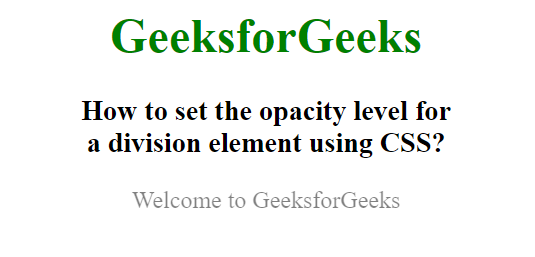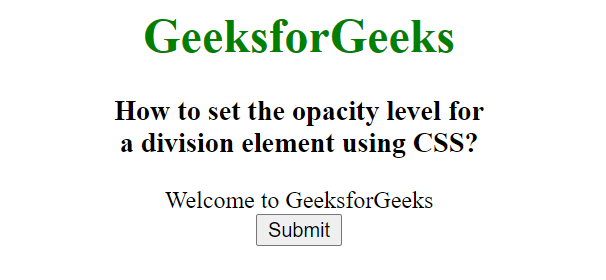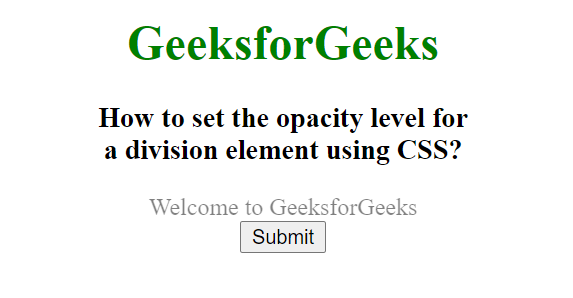Related Articles
How to set the opacity level for a division element using CSS ?
• Last Updated : 31 Dec, 2020

In this article, we will set the opacity of an element using CSS. Opacity is the property of an element which describes the transparency of the element.

The opacity property is used to describe the transparency of the element. The value of opacity lies between 0.0 to 1.0 where a low value represents high transparent and high value represents low transparent. The percentage of opacity is calculated as Opacity% = Opacity * 100.

Example 1:

## HTML

 ```<``html` `lang``=``"en"``>`` ` `<``head``>``    ``<``title``>``        ``How to set the opacity level for``        ``a division element using CSS?``    ````    ``<``style``>``        ``body {``            ``text-align: center;``        ``}`` ` `        ``h1 {``            ``color: green;``        ``}`` ` `        ``.GFG {``            ``opacity: 0.5;``        ``}``    `````` ` `<``body``>``    ``<``h1``>GeeksforGeeks`` ` `    ``<``h3``>``        ``How to set the opacity level for``        ``<``br``>a division element using CSS?``    ```` ` `    ``<``div` `class``=``"GFG"``>``        ``Welcome to GeeksforGeeks``    `````` ` ``

Output:Example 2:

## HTML

 ```<``html` `lang``=``"en"``>`` ` `<``head``>``    ``<``title``>``        ``How to set the opacity level for``        ``a division element using CSS?``    ````    ``<``style``>``        ``body {``            ``text-align: center;``        ``}`` ` `        ``h1 {``            ``color: green;``        ``}``    ```` ` `    ``<``script``>``        ``function fun() {``            ``document.getElementById("GFG")``                ``.style.opacity = "0.5";``        ``} ``    `````` ` `<``body``>``    ``<``h1``>GeeksforGeeks`` ` `    ``<``h3``>``        ``How to set the opacity level for``        ``<``br``>a division element using CSS?``    ```` ` `    ``<``div` `id``=``"GFG"``>``        ``Welcome to GeeksforGeeks``    ```` ` `    ``<``button` `type``=``"button"` `onclick``=``"fun()"``>``        ``Submit``    `````` ` ``

Output:

Before Button Click:After Button Click:My Personal Notes arrow_drop_up